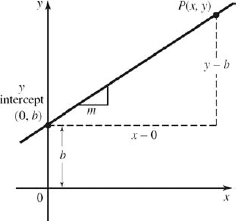### 22.2. Equation of a Straight Line

We said earlier that one value of analytic geometry was the ability to write an equation for a geometric figure. Then we could analyze that figure using algebra. Here we will write an equation for a geometric figure, the straight line. Such an equation would relate y to x for any point on the figure, so that given one coordinate, we could find the other from the equation.

#### 22.2.1. Slope-Intercept Form

We covered the slope-intercept form of the equation of a straight line in our chapter on graphing, and we will repeat some of that material here. We will also show a few other forms of the straight line equation.

One way we can get such an equation is from our definition of slope. Let P(x, y) be any point on a line, Fig. 22-20, and let the y intercept, (0, b) be a second point on the line. Between P and the y intercept, the rise is (yb) and the run is (x − 0). The slope m of the line is thenSimplifying, we have mx = yb, or##### Figure 22.20. FIGURE 22-20This is called the slope-intercept form of the equation of a straight line because the slope m and the y intercept b are easily identified once the equation is in this form. For example, in the ...

Get Technical Mathematics, Sixth Edition now with the O’Reilly learning platform.

O’Reilly members experience live online training, plus books, videos, and digital content from nearly 200 publishers.